JMSLTM Numerical Library 7.2.0
com.imsl.stat

## Class AutoARIMA

• All Implemented Interfaces:
Serializable, Cloneable

```public class AutoARIMA
extends Object
implements Serializable, Cloneable```
Automatically identifies time series outliers, determines parameters of a multiplicative seasonal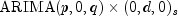model and produces forecasts that incorporate the effects of outliers whose effects persist beyond the end of the series.

Class `AutoARIMA` determines the parameters of a multiplicative seasonalmodel, and then uses the fitted model to identify outliers and prepare forecasts. The order of this model can be specified or automatically determined through use of an overloaded `compute` method. Potential missing values in the time series are estimated prior to the parameter and outlier computations.

Themodel handled by class `AutoARIMA` has the following form: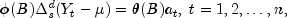where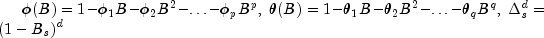and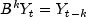It is assumed that all roots of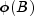and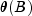lie outside the unit circle. Clearly, if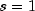the model reduces to the traditional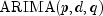model.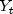is the unobserved, outlier-free time series with mean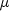, and white noise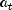. This model is referred to as the underlying, outlier-free model. Class ``` AutoARIMA``` does not assume that this series is observable. It assumes that the observed values might be contaminated by one or more outliers, whose effects are added to the underlying outlier-free series: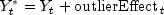Outlier identification uses the algorithm developed by Chen and Liu (1993). Outliers are classified into 1 of 5 types:

1. innovational
3. level shift
4. temporary change and
5. unable to identify

Once the model parameters are estimated and the outliers are identified, class `AutoARIMA` estimates, the outlier-free series representation of the data, by removing the estimated outlier effects. Parameter estimation and outlier detection are based on methods from class `ARMAOutlierIdentification`.

Using the information about the adjustedmodel and the removed outliers, forecasts are then prepared for the outlier-free series. Outlier effects are added to these forecasts to produce a forecast for the observed series,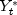. If there are no outliers, then the forecasts for the outlier-free series and the observed series will be identical.

Model selection techniques

Users have an option of either specifying specific values for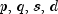or have class `AutoARIMA` automatically select best fit values. Model selection can be conducted in one of three ways listed below depending upon which `compute` method of class ``` AutoARIMA``` is invoked.

Technique 1: Automatic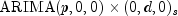Selection

This technique, chosen by use of method `compute(int maxlag)`, tries to fit a model of the form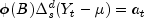to the outlier free series.

It initially searches for the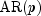representation with minimum value of the chosen information criterion (AIC, AICC or BIC) for the noisy data, where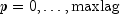.

If the user calls methods `setPeriods` and ``` setDifferenceOrders``` prior to invoking the `compute` method, then the values in arrays `periods` and `orders` are included in the search to find an optimumrepresentation of the series. Here, every possible combination of values for s, d in `periods` and `orders`, respectively, are examined. The best found model order is then used as input for the parameter and outlier detection routine.

The optimum values for p, q, s and d are returned through method `getOptimumModelOrder`.

Technique 2: Grid Search

This technique, chosen by means of method ```compute(int[] arOrders, int[] maOrders)```, conducts a grid search for p and q using all possible combinations of candidate values in `arOrders` and `maOrders`.

If methods `setPeriods` and `setDifferenceOrders` are called prior to invoking the `compute` method, then the grid search is extended to include the candidate values for s and d given in arrays `periods` and `orders`, respectively.

If method `setDifferenceOrders` is not called prior to `compute`, then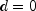by default, and therefore no seasonal adjustment is attempted. The grid search is then restricted to searching for optimum values of p and q only.

The optimum values for p, q, s and d are contained in the array returned by method `getOptimumModelOrder`.

Technique 3: SpecifiedModel

In the third technique, selectable by means of method ```compute(int p, int q, int s, int d)```, specific values for p, q, s and d are given. This technique has essentially the same functionality as class `ARMAOutlierIdentification` but with the additional option of missing value estimation.

Outliers

The algorithm of Chen and Liu (1993) is used to identify outliers. The number of outliers identified is returned via method ``` getNumberOfOutliers```. Both the time and classification for these outliers are contained in the matrix returned by method ``` getOutlierStatistics```. Outliers are classified into one of five categories based upon the standardized statistic for each outlier type. The time at which the outlier occurred is given in the first column of the returned matrix. The outlier identifier returned in the second column is according to the descriptions in the following table:

 Outlier Identifier Name General Description `INNOVATIONAL` Innovational Outlier (IO) Innovational outliers persist. That is, there is an initial impact at the time the outlier occurs. This effect continues in a lagged fashion with all future observations. The lag coefficients are determined by the coefficients of the underlyingmodel. `ADDITIVE` Additive Outlier (AO) Additive outliers do not persist. As the name implies, an additive outlier affects only the observation at the time the outlier occurs. Hence additive outliers have no effect on future forecasts. `LEVEL_SHIFT` Level Shift (LS) Level shift outliers persist. They have the effect of either raising or lowering the mean of the series starting at the time the outlier occurs. This shift in the mean is abrupt and permanent. `TEMPORARY_CHANGE` Temporary Change (TC) Temporary change outliers persist and are similar to level shift outliers with one major exception. Like level shift outliers, there is an abrupt change in the mean of the series at the time this outlier occurs. However, unlike level shift outliers, this shift is not permanent. The TC outlier gradually decays, eventually bringing the mean of the series back to its original value. The rate of this decay is modeled using method `setDelta`. The default of `delta = 0.7` is the value recommended for general use by Chen and Liu (1993). `UNABLE_TO_IDENTIFY` Unable to Identify (UI) If an outlier is identified as the last observation, then the algorithm is unable to determine the outlier's classification. For forecasting, a UI outlier is treated as an IO outlier. That is, its effect is lagged into the forecasts.

Except for additive outliers (AO), the effect of an outlier persists to observations following that outlier. Forecasts produced by methods of class `AutoARIMA` take this into account.

For more information on forecasting an outlier contaminated series, see the description of class `ARMAOutlierIdentification`.

Example 1, Example 2, Example 3, Serialized Form
• ### Nested Class Summary

Nested Classes
Modifier and Type Class and Description
`static class ` `AutoARIMA.NoAcceptableModelFoundException`
No appropriate ARIMA model could be found.
• ### Field Summary

Fields
Modifier and Type Field and Description
`static int` `ADDITIVE`
Indicates detection of an additive outlier.
`static int` `AIC`
Indicates that Akaike's information criterion (AIC) is used in the optimum model determination.
`static int` `AICC`
Indicates that Akaike's corrected information criterion (AICC) is used in the optimum model determination.
`static int` `BIC`
Indicates that the Bayesian information criterion (BIC) is used in the optimum model determination.
`static int` `INNOVATIONAL`
Indicates detection of an innovational outlier.
`static int` `LEVEL_SHIFT`
Indicates detection of a level shift outlier.
`static int` `TEMPORARY_CHANGE`
Indicates detection of a temporary change outlier.
`static int` `UNABLE_TO_IDENTIFY`
Indicates detection of an outlier that cannnot be categorized.
• ### Constructor Summary

Constructors
Constructor and Description
```AutoARIMA(int[] times, double[] x)```
Constructor for `AutoARIMA`.
• ### Method Summary

Methods
Modifier and Type Method and Description
`void` `compute(int maxlag)`
Estimates potential missing values, detects and determines outliers and simultaneously fits an optimum model from a set of differentmodels to the outlier free time series.
`void` ```compute(int[] arOrders, int[] maOrders)```
Estimates potential missing values, detects and determines outliers and simultaneously fits an optimum model from a set of differentmodels to the outlier free time series.
`void` ```compute(int p, int q, int s, int d)```
Estimates potential missing values, detects and determines outliers and simultaneously fits anmodel to the outlier free time series.
`void` `forecast(int nForecast)`
Computes forecasts, associated probability limits and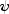weights for the given outlier contaminated time series.
`double` `getAIC()`
Returns Akaike's information criterion (AIC) for the optimum model.
`double` `getAICC()`
Returns Akaike's Corrected Information Criterion (AICC) for the optimum model.
`double[]` `getAR()`
Returns the final autoregressive parameter estimates of the optimum model.
`double` `getBIC()`
Returns the Bayesian Information Criterion (BIC) for the optimum model.
`int[]` `getCompleteTimes()`
Returns all time points at which the original series was observed, including values for times with missing values in `x`.
`double[]` `getCompleteTimeSeries()`
Returns the original series with potentially missing values replaced by estimates.
`double` `getConstant()`
Returns the constant parameter estimate for the optimum model.
`double[]` `getDeviations()`
Returns the deviations used for calculating the forecast confidence limits.
`double[]` `getForecast()`
Returns forecasts for the original outlier contaminated series.
`double[]` `getMA()`
Returns the final moving average parameter estimates of the optimum model.
`int` `getNumberOfOutliers()`
Returns the number of outliers detected.
`int[]` `getOptimumModelOrder()`
Returns the order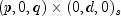of the optimum model.
`double[]` `getOutlierFreeForecast()`
Returns forecasts for the outlier free series.
`double[]` `getOutlierFreeSeries()`
Returns the outlier free series.
`int[][]` `getOutlierStatistics()`
Returns the outlier statistics.
`double[]` `getPsiWeights()`
Returns theweights of the infinite order moving average form of the model.
`double[]` `getResiduals()`
Returns the residuals.
`double` `getResidualStandardError()`
Returns the residual standard error of the outlier free series.
`void` `setAccuracyTolerance(double epsilon)`
Sets the tolerance value controlling the accuracy of the parameter estimates.
`void` `setConfidence(double confidence)`
Sets the confidence level for calculating confidence limit deviations returned by `getDeviations`.
`void` `setCriticalValue(double critical)`
Sets the critical value used as a threshold during outlier detection.
`void` `setDelta(double delta)`
Sets the dampening effect parameter.
`void` `setDifferenceOrders(int[] orders)`
Defines the orders of the periodic differences used in the determination of the optimum model.
`void` `setMaximumARLag(int maxARLag)`
Defines the maximum AR lag used in the determination of the optimum (s,d) combination of method `compute(int[] arOrders, int[] maOrders)`.
`void` `setModelSelectionCriterion(int infoCriterion)`
Sets the model selection criterion.
`void` `setPeriods(int[] periods)`
Defines the periods used in the determination of the optimum model.
`void` `setRelativeError(double relativeError)`
Sets the stopping criterion for use in the nonlinear equation solver.
• ### Methods inherited from class java.lang.Object

`clone, equals, finalize, getClass, hashCode, notify, notifyAll, toString, wait, wait, wait`
• ### Field Detail

`public static final int ADDITIVE`
Indicates detection of an additive outlier.
Constant Field Values
• #### AIC

`public static final int AIC`
Indicates that Akaike's information criterion (AIC) is used in the optimum model determination.
Constant Field Values
• #### AICC

`public static final int AICC`
Indicates that Akaike's corrected information criterion (AICC) is used in the optimum model determination.
Constant Field Values
• #### BIC

`public static final int BIC`
Indicates that the Bayesian information criterion (BIC) is used in the optimum model determination.
Constant Field Values
• #### INNOVATIONAL

`public static final int INNOVATIONAL`
Indicates detection of an innovational outlier.
Constant Field Values
• #### LEVEL_SHIFT

`public static final int LEVEL_SHIFT`
Indicates detection of a level shift outlier.
Constant Field Values
• #### TEMPORARY_CHANGE

`public static final int TEMPORARY_CHANGE`
Indicates detection of a temporary change outlier.
Constant Field Values
• #### UNABLE_TO_IDENTIFY

`public static final int UNABLE_TO_IDENTIFY`
Indicates detection of an outlier that cannnot be categorized.
Constant Field Values
• ### Constructor Detail

• #### AutoARIMA

```public AutoARIMA(int[] times,
double[] x)```
Constructor for `AutoARIMA`.
Parameters:
`times` - an `int` array of length `nObs`, where `nObs` is the number of observed time series values, containing the time points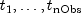at which the time series was observed. It is required thatare in strictly increasing order. Times for missing values are identified as non-incremental gaps in this series. A gap of missing values in `x` is assumed if the difference between two consecutive values is greater than 1, i.e.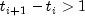. The difference is the number of missing values in the gap.
`x` - a `double` array containing the observations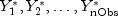at the times given in array `times`. This series can contain outliers and missing observations.
• ### Method Detail

• #### compute

```public final void compute(int maxlag)
throws ARMA.MatrixSingularException,
ARMA.TooManyCallsException,
ARMA.IncreaseErrRelException,
ARMA.NewInitialGuessException,
ARMA.IllConditionedException,
ARMA.TooManyITNException,
ARMA.TooManyFcnEvalException,
ARMA.TooManyJacobianEvalException,
ARAutoUnivariate.TriangularMatrixSingularException,
ARMAMaxLikelihood.NonInvertibleException,
ARMAMaxLikelihood.NonStationaryException,
ARMAMaxLikelihood.InitialMAException,
ZeroPolynomial.DidNotConvergeException,
SingularMatrixException,
Cholesky.NotSPDException,
AutoARIMA.NoAcceptableModelFoundException```
Estimates potential missing values, detects and determines outliers and simultaneously fits an optimum model from a set of differentmodels to the outlier free time series.
Parameters:
`maxlag` - the maximum value for p allowed when fittingmodels to the given series,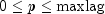. It is required that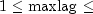`x.length`. The optimummodel is determined according to the model selection criterion chosen by the user, see method ``` setModelSelectionCriterion```.
Throws:
`ARMA.MatrixSingularException` - is thrown if the input matrix is singular.
`ARMA.TooManyCallsException` - is thrown if the number of calls to the function has exceeded the maximum number of iterations times the number of moving average (MA) parameters + 1.
`ARMA.IncreaseErrRelException` - is thrown if the bound for the relative error is too small.
`ARMA.NewInitialGuessException` - is thrown if the iteration has not made good progress.
`ARMA.IllConditionedException` - is thrown if the problem is ill-conditioned.
`ARMA.TooManyITNException` - is thrown if the maximum number of iterations is exceeded.
`ARMA.TooManyFcnEvalException` - is thrown if the maximum number of function evaluations is exceeded.
`ARMA.TooManyJacobianEvalException` - is thrown if the maximum number of Jacobian evaluations is exceeded.
`ARAutoUnivariate.TriangularMatrixSingularException` - is thrown if the input matrix to ARAutoUnivariate is singular.
`ARMAMaxLikelihood.NonInvertibleException` - is thrown if the intermediate or final maximum likelihood estimates for the time series are noninvertible.
`ARMAMaxLikelihood.NonStationaryException` - is thrown if the intermediate or final maximum likelihood estimates for the time series are nonstationary.
`ARMAMaxLikelihood.InitialMAException` - is thrown if the initial values provided for the moving average terms are noninvertible. In this case, ```ARMAMaxLikelihood ``` terminates and does not compute the time series estimates.
`ZeroPolynomial.DidNotConvergeException` - is thrown if the algorithm computing the roots of the AR- or MA- polynomial does not converge.
`SingularMatrixException` - is thrown if during the computation of a small perturbation of the matrix product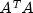, it is found that A, the matrix used in the determination of theweights, is singular.
`Cholesky.NotSPDException` - is thrown if during the computation of a small perturbation of the matrix product, it is found that A, the matrix used in the determination of theweights, is not positive definite.
`AutoARIMA.NoAcceptableModelFoundException` - is thrown if no appropriate ARIMA model for the given time series could be found.
• #### compute

```public final void compute(int[] arOrders,
int[] maOrders)
throws ARMA.MatrixSingularException,
ARMA.TooManyCallsException,
ARMA.IncreaseErrRelException,
ARMA.NewInitialGuessException,
ARMA.IllConditionedException,
ARMA.TooManyITNException,
ARMA.TooManyFcnEvalException,
ARMA.TooManyJacobianEvalException,
ARAutoUnivariate.TriangularMatrixSingularException,
ARMAMaxLikelihood.NonInvertibleException,
ARMAMaxLikelihood.NonStationaryException,
ARMAMaxLikelihood.InitialMAException,
ZeroPolynomial.DidNotConvergeException,
SingularMatrixException,
Cholesky.NotSPDException,
AutoARIMA.NoAcceptableModelFoundException```
Estimates potential missing values, detects and determines outliers and simultaneously fits an optimum model from a set of differentmodels to the outlier free time series.
Parameters:
`arOrders` - an `int` array containing all possible AR orders to consider in the optimum model search. It is required that all values in `arOrders` are greater than or equal to zero.
`maOrders` - an `int` array containing all possible MA orders to consider in the optimum model search. It is required that all values in `maOrders` are greater than or equal to zero.
Throws:
`ARMA.MatrixSingularException` - is thrown if the input matrix is singular.
`ARMA.TooManyCallsException` - is thrown if the number of calls to the function has exceeded the maximum number of iterations times the number of moving average (MA) parameters + 1.
`ARMA.IncreaseErrRelException` - is thrown if the bound for the relative error is too small.
`ARMA.NewInitialGuessException` - is thrown if the iteration has not made good progress.
`ARMA.IllConditionedException` - is thrown if the problem is ill-conditioned.
`ARMA.TooManyITNException` - is thrown if the maximum number of iterations is exceeded.
`ARMA.TooManyFcnEvalException` - is thrown if the maximum number of function evaluations is exceeded.
`ARMA.TooManyJacobianEvalException` - is thrown if the maximum number of Jacobian evaluations is exceeded.
`ARAutoUnivariate.TriangularMatrixSingularException` - is thrown if the input matrix to ARAutoUnivariate is singular.
`ARMAMaxLikelihood.NonInvertibleException` - is thrown if the intermediate or final maximum likelihood estimates for the time series are noninvertible.
`ARMAMaxLikelihood.NonStationaryException` - is thrown if the intermediate or final maximum likelihood estimates for the time series are nonstationary.
`ARMAMaxLikelihood.InitialMAException` - is thrown if the initial values provided for the moving average terms are noninvertible. In this case, ```ARMAMaxLikelihood ``` terminates and does not compute the time series estimates.
`ZeroPolynomial.DidNotConvergeException` - is thrown if the algorithm computing the roots of the AR- or MA- polynomial does not converge.
`SingularMatrixException` - is thrown if during the computation of a small perturbation of the matrix product, it is found that A, the matrix used in the determination of theweights, is singular.
`Cholesky.NotSPDException` - is thrown if during the computation of a small perturbation of the matrix product, it is found that A, the matrix used in the determination of theweights, is not positive definite.
`AutoARIMA.NoAcceptableModelFoundException` - is thrown if no appropriate ARIMA model for the given time series could be found.
• #### compute

```public final void compute(int p,
int q,
int s,
int d)
throws ARMA.MatrixSingularException,
ARMA.TooManyCallsException,
ARMA.IncreaseErrRelException,
ARMA.NewInitialGuessException,
ARMA.IllConditionedException,
ARMA.TooManyITNException,
ARMA.TooManyFcnEvalException,
ARMA.TooManyJacobianEvalException,
ARAutoUnivariate.TriangularMatrixSingularException,
ARMAMaxLikelihood.NonInvertibleException,
ARMAMaxLikelihood.NonStationaryException,
ARMAMaxLikelihood.InitialMAException,
ZeroPolynomial.DidNotConvergeException,
SingularMatrixException,
Cholesky.NotSPDException,
AutoARIMA.NoAcceptableModelFoundException```
Estimates potential missing values, detects and determines outliers and simultaneously fits anmodel to the outlier free time series.
Parameters:
`p` - a non-negative scalar `int`, the order of the AR part of the model.
`q` - a non-negative scalar `int`, the order of the MA part of the model.
`s` - a positive scalar `int`, the period of the difference used in the model.
`d` - a non-negative scalar `int`, the order of the difference used in the model.
Throws:
`ARMA.MatrixSingularException` - is thrown if the input matrix is singular.
`ARMA.TooManyCallsException` - is thrown if the number of calls to the function has exceeded the maximum number of iterations times the number of moving average (MA) parameters + 1.
`ARMA.IncreaseErrRelException` - is thrown if the bound for the relative error is too small.
`ARMA.NewInitialGuessException` - is thrown if the iteration has not made good progress.
`ARMA.IllConditionedException` - is thrown if the problem is ill-conditioned.
`ARMA.TooManyITNException` - is thrown if the maximum number of iterations is exceeded.
`ARMA.TooManyFcnEvalException` - is thrown if the maximum number of function evaluations is exceeded.
`ARMA.TooManyJacobianEvalException` - is thrown if the maximum number of Jacobian evaluations is exceeded.
`ARAutoUnivariate.TriangularMatrixSingularException` - is thrown if the input matrix to ARAutoUnivariate is singular.
`ARMAMaxLikelihood.NonInvertibleException` - is thrown if the intermediate or final maximum likelihood estimates for the time series are noninvertible.
`ARMAMaxLikelihood.NonStationaryException` - is thrown if the intermediate or final maximum likelihood estimates for the time series are nonstationary.
`ARMAMaxLikelihood.InitialMAException` - is thrown if the initial values provided for the moving average terms are noninvertible. In this case, ```ARMAMaxLikelihood ``` terminates and does not compute the time series estimates.
`ZeroPolynomial.DidNotConvergeException` - is thrown if the algorithm computing the roots of the AR- or MA- polynomial does not converge.
`SingularMatrixException` - is thrown if during the computation of a small perturbation of the matrix product, it is found that A, the matrix used in the determination of theweights, is singular.
`Cholesky.NotSPDException` - is thrown if during the computation of a small perturbation of the matrix product, it is found that A, the matrix used in the determination of theweights, is not positive definite.
`AutoARIMA.NoAcceptableModelFoundException` - is thrown if no appropriate ARIMA model for the given time series could be found.
• #### forecast

`public final void forecast(int nForecast)`
Computes forecasts, associated probability limits andweights for the given outlier contaminated time series. Note that one of the `compute` methods must be invoked first before invoking this method. Otherwise, the method throws an `IllegalStateException` exception.
Parameters:
`nForecast` - an `int` scalar representing the number of forecasts that will be computed. `nForecast` must be greater than 0. Forecast origin is the time point of the last observed value in the time series,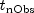. Forecasts are computed for lead times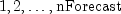, i.e. time points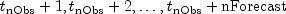.
• #### getAIC

`public double getAIC()`
Returns Akaike's information criterion (AIC) for the optimum model.
Returns:
a `double` scalar containing Akaike's information criterion (AIC) for the optimum outlier free series. One of the `compute` methods must be called before invoking this method. Otherwise, an `IllegalStateException` exception is thrown.
• #### getAICC

`public double getAICC()`
Returns Akaike's Corrected Information Criterion (AICC) for the optimum model.
Returns:
a `double` scalar containing Akaike's Corrected Information Criterion (AICC) for the optimum outlier free series. One of the `compute` methods must be called before invoking this method. Otherwise, an ```IllegalStateException ``` exception is thrown.
• #### getAR

`public double[] getAR()`
Returns the final autoregressive parameter estimates of the optimum model. Note that one of the `compute` methods must be invoked first before invoking this method. Otherwise, the method throws an ``` IllegalStateException``` exception.
Returns:
a `double` array containing the final autoregressive parameter estimates. If the optimum model has no AR component then a zero-length array is returned.
• #### getBIC

`public double getBIC()`
Returns the Bayesian Information Criterion (BIC) for the optimum model.
Returns:
a `double` scalar containing the Bayesian Information Criterion (BIC) for the optimum outlier free series. One of the `compute` methods must be called before invoking this method. Otherwise, an `IllegalStateException` exception is thrown.
• #### getCompleteTimes

`public int[] getCompleteTimes()`
Returns all time points at which the original series was observed, including values for times with missing values in `x`.
Returns:
an `int` array of length `times[times.length-1]-times+1` containing the times at which the time series (including missing values) was observed. One of the `compute` methods must be called before invoking this method. Otherwise, an `IllegalStateException` exception is thrown.
• #### getCompleteTimeSeries

`public double[] getCompleteTimeSeries()`
Returns the original series with potentially missing values replaced by estimates.
Returns:
a `double` array containing the original time series with missing values replaced by estimates. One of the ``` compute``` methods must be called before invoking this method. Otherwise, an `IllegalStateException` exception is thrown.
• #### getConstant

`public double getConstant()`
Returns the constant parameter estimate for the optimum model. Note that one of the `compute` methods must be invoked first before invoking this method. Otherwise, the method throws an ``` IllegalStateException``` exception.
Returns:
a `double` scalar containing the constant parameter estimate for the optimum model.
• #### getDeviations

`public double[] getDeviations()`
Returns the deviations used for calculating the forecast confidence limits.
Returns:
a `double` array of length `nForecast` containing the deviations from each forecast for calculating forecast confidence intervals. The confidence level is specified in `confidence`. Method `forecast` must be invoked before this method is called. Otherwise, an `IllegalStateException` exception is thrown. By default, `confidence` = 0.95.
• #### getForecast

`public double[] getForecast()`
Returns forecasts for the original outlier contaminated series.
Returns:
a `double` array of length `nForecast` containing the forecasts for the original series. Forecast origin is the time point of the last observed value in the time series,. Forecasts are returned for lead times, i.e. time points. Method ` forecast` must be invoked before this method is called. Otherwise, an `IllegalStateException` exception is thrown.
• #### getMA

`public double[] getMA()`
Returns the final moving average parameter estimates of the optimum model. Note that one of the `compute` methods must be invoked first before invoking this method. Otherwise, the method throws an ``` IllegalStateException``` exception.
Returns:
a `double` array containing the final moving average parameter estimates. If the optimum model has no MA component then a zero-length array is returned.
• #### getNumberOfOutliers

`public int getNumberOfOutliers()`
Returns the number of outliers detected.
Returns:
an `int` scalar containing the number of outliers detected. The `compute` method must be invoked first before invoking this method. Otherwise, the method throws an `IllegalStateException` exception.
• #### getOptimumModelOrder

`public int[] getOptimumModelOrder()`
Returns the orderof the optimum model. Note that one of the `compute` methods must be invoked first before calling this method. Otherwise, an ``` IllegalStateException``` exception is thrown.
Returns:
an `int` array of length 4 containing the values p, q, s and d for the optimum model.
• #### getOutlierFreeForecast

`public double[] getOutlierFreeForecast()`
Returns forecasts for the outlier free series.
Returns:
a `double` array of length `nForecast` containing the forecasts for the outlier free series. Forecast origin is the time point of the last observed value in the time series,. Forecasts are returned for lead times, i.e. time points. Method `forecast` must be invoked before this method is called. Otherwise, an `IllegalStateException` exception is thrown.
• #### getOutlierFreeSeries

`public double[] getOutlierFreeSeries()`
Returns the outlier free series.
Returns:
a `double` array containing the original time series with estimated missing values after removal of any outlier effects. One of the `compute` methods must be called before invoking this method. Otherwise, an ``` IllegalStateException``` exception is thrown.
• #### getOutlierStatistics

`public int[][] getOutlierStatistics()`
Returns the outlier statistics.
Returns:
a `double` array of length `nOutliers` by 2, where `nOutliers` is the number of detected outliers, containing the outlier statistics. The first column contains the time at which the outlier was observed (time ranging from ``` times``` to `times[times.length - 1]`) and the second column contains an identifier indicating the type of outlier observed. Outlier types fall into one of five categories:  Identifier Outlier type `INNOVATIONAL` Innovational Outliers (IO) `ADDITIVE` Additive Outliers (AO) `LEVEL_SHIFT` Level Shift Outliers (LS) `TEMPORARY_CHANGE` Temporary Change Outliers (TC) `UNABLE_TO_IDENTIFY` Unable to Identify (UI)

If the number of detected outliers equals zero, then an array of length zero is returned.

• #### getPsiWeights

`public double[] getPsiWeights()`
Returns theweights of the infinite order moving average form of the model.
Returns:
a `double` array of length `nForecast` containing theweights of the infinite order moving average form of the optimum model for the outlier free series. Method `forecast` must be invoked before this method is called. Otherwise, an ``` IllegalStateException``` exception is thrown.
• #### getResiduals

`public double[] getResiduals()`
Returns the residuals. Note that one of the `compute` methods must be invoked first before invoking this method. Otherwise, ``` getResiduals``` throws an `IllegalStateException` exception.
Returns:
a `double` array containing the residuals for the outlier and gap free series at the final parameter estimation point.
• #### getResidualStandardError

`public double getResidualStandardError()`
Returns the residual standard error of the outlier free series. Note that one of the `compute` methods must be invoked first before calling this method. Otherwise, an `IllegalStateException` exception is thrown.
Returns:
a `double` scalar containing the standard error of the outlier free series.
• #### setAccuracyTolerance

`public void setAccuracyTolerance(double epsilon)`
Sets the tolerance value controlling the accuracy of the parameter estimates.
Parameters:
`epsilon` - a `double` scalar, a positive tolerance value controlling the accuracy of parameter estimates during outlier detection. Default: `epsilon = 0.001`.
• #### setConfidence

`public void setConfidence(double confidence)`
Sets the confidence level for calculating confidence limit deviations returned by `getDeviations`.
Parameters:
`confidence` - a `double` scalar specifying the confidence level used in computing forecast confidence intervals. Typical choices for ``` confidence``` are 0.90, 0.95, and 0.99. ``` confidence``` must be greater than 0.0 and less than 1.0. Default: `confidence = 0.95`.
• #### setCriticalValue

`public void setCriticalValue(double critical)`
Sets the critical value used as a threshold during outlier detection.
Parameters:
`critical` - a `double` scalar, the critical value used as a threshold for the statistics used in the outlier detection. `critical` must be greater than zero. Default: `critical = 3.0`.
• #### setDelta

`public void setDelta(double delta)`
Sets the dampening effect parameter.
Parameters:
`delta` - a `double` scalar, the dampening effect parameter used in the detection of a Temporary Change Outlier (TC). `delta` must be greater than 0 and less than 1. Default: `delta = 0.7`.
• #### setDifferenceOrders

`public void setDifferenceOrders(int[] orders)`
Defines the orders of the periodic differences used in the determination of the optimum model.
Parameters:
`orders` - an `int` array containing all possible orders for each difference given in `periods`. All elements in `orders` must be non-negative. By default, `orders` is a one-element array with `orders` = 0.
• #### setMaximumARLag

`public void setMaximumARLag(int maxARLag)`
Defines the maximum AR lag used in the determination of the optimum (s,d) combination of method `compute(int[] arOrders, int[] maOrders)`.
Parameters:
`maxARLag` - a scalar `int`, the maximum AR lag used in the computation of the optimum (s,d) combination for method `compute(int[] arOrders, int[] maOrders)`. It is required that `maxARLag` is greater than zero and smaller than the original series after replacement of potential missing values. By default, `maxARLag` = 10.
• #### setModelSelectionCriterion

`public void setModelSelectionCriterion(int infoCriterion)`
Sets the model selection criterion.
Parameters:
`infoCriterion` - an `int` scalar indicating the model selection criterion used in the search for the optimum model.  infoCriterion Model selection criterion `AIC` Akaike's information criterion (AIC) `AICC` Akaike's corrected information criterion (AICC) `BIC` Bayesian information criterion (BIC)

By default, AIC is chosen.

• #### setPeriods

`public void setPeriods(int[] periods)`
Defines the periods used in the determination of the optimum model.
Parameters:
`periods` - an `int` array containing all possible periods that can be applied to the original series after insertion of missing values. All elements of `periods` must be positive. By default, `periods` is a one-element array with `periods` = 1;
• #### setRelativeError

`public void setRelativeError(double relativeError)`
Sets the stopping criterion for use in the nonlinear equation solver.
Parameters:
`relativeError` - a `double` positive scalar containing the stopping criterion for use in the nonlinear equation solver used in the least-squares algorithm. Default: ```relativeError = 2.2204460492503131e-012```.
JMSLTM Numerical Library 7.2.0